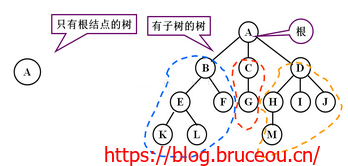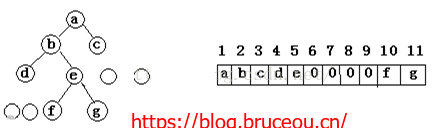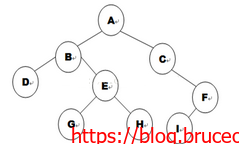• 欢迎访问BruceOu的博客，推荐使用最新版火狐浏览器和Chrome浏览器访问本网站
• 如果您觉得本站非常有看点，那么赶紧使用Ctrl+D 收藏本章吧

# 《数据结构与算法》第8章 树 (C语言)

5个月前 (07-04) 150次浏览## 8.1树的概念

1）有且仅有一个特定的称为根（root）的节点；
2）其余的节点可以分为m(m>=0)个互不相交的有限结合T1、T2、…、Tm，其中每一个集合又是一棵树，并成为其根的子数（Subtree）。## 8.2二叉树1、二叉树的顺序存储结构

1）当i > 1（不是根节点）时，有父节点，其父节点编号为i / 2；
2）当2 * i <= n 时，有左子树，其编号为2 * i，否则没有左子树，本身是叶节点；
3）当2 * i + 1 < = n时，有右子树，其编号为2 * i + 1，否则没有右子树；2、二叉树的链式存储
1）链式存储结构：

typedef int data_t;
typedef struct node_t //定义二叉树节点的内部结构
{
data_t data;
struct node_t *lchild,*rchild; //指向左孩子和右孩子的指针；
}bitree_t;
bitree_t *root; //定义指向二叉树的指针


2）链式二叉树的创建data_t a[] = {0,'a','b','c','d','e',0,'f',0,0,'g','h',0,0,'i'};


data_t a[] = {0,'a','b','c','d','e',0,'f',0,0,'g','h',0,0,'i'};
bitree_t *CreateBitree(int i, data_t a[], int n)
{
bitree_t *root;
int j;
root = (bitree_t *)malloc(sizeof(bitree_t));
root->data = a[i];

j = 2 * i;
if(j <= n && a[j] != '0')
{
root->lchild = CreateBitree(j, a, n); //创建
}
else
{
root->lchild = NULL;
}

j = 2 * i + 1;
if(j <= n && a[j] != '0')
{
root->rchild = CreateBitree(j, a, n);
}
else
{
root->rchild = NULL;
}
return root;
}3、二叉树的遍历

1）先序遍历：先访问树根，再访问左子树，最后访问右子树；
2）中序遍历：先访问左子树，再访问树根，最后访问右子树；
3）后序遍历：先访问左子树，再访问右子树，最后访问树根；

void PREORDER(bitree_t *r)
{
if(r == NULL)
return;
printf("%c ",r->data);
PREORDER(r->lchild);
PREORDER(r->rchild);
}


void INORDER(bitree_t *r)
{
if(r == NULL)
return;
INORDER(r->lchild);
printf("%c ",r->data);
INORDER(r->rchild);
}


void POSTORDER(bitree_t *r)
{
if(r == NULL)
return;
POSTORDER(r->lchild);
POSTORDER(r->rchild);
printf("%c ",r->data);
}


int main()
{
bitree_t *tree;

printf("Begin creating the tree!\n");
tree = CreateBitree(1,a,sizeof(a)/sizeof(data_t) - 1);
printf("Finish!\n");

printf("Preorder traversal:\n");
PREORDER(tree);

printf("\nInorder traversal:\n");
INORDER(tree);

printf("\nPostorder traversal:\n");
POSTORDER(tree);
printf("\n");

return 0;
}


\$ ./bitree
Begin creating the tree!
Finish!
Preorder traversal:
a b d e g h c f i
Inorder traversal:
d b g e h a c i f
Postorder traversal:
d g h e b i f c a



### 资源获取方法

1.长按下面二维码，关注公众号[嵌入式实验楼]
2.在公众号回复关键词[Data Structures and Algorithms]获取资料提取码BrueceOu , 版权所有丨如未注明 , 均为原创丨本网站采用BY-NC-SA协议进行授权

[支付宝]• 版权声明

本站的文章和资源来自互联网或者站长
的原创，按照 CC BY -NC -SA 3.0 CN
协议发布和共享，转载或引用本站文章
应遵循相同协议。如果有侵犯版权的资
源请尽快联系站长，我们会在24h内删
除有争议的资源。
• 网站驱动

• 友情链接

• 支持主题